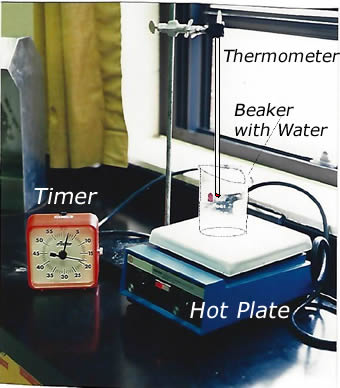#### Introduction

• The Scientific Method
• Models, Theories, Laws
• Observations

#### Some basic scientific terms you need to be familiar with:

• Science is the body of knowledge that explains the nature of matter, and how energy and matter interact.
• Technology uses scientific discoveries to invent devices and processes to advance the progress of society. Technology is applied Science.
• Matter is all that is around us. Mater is what the universe is made of.  Therefore, one could define matter as "anything that has mass and occupies volume".
• Mass is the amount of substance in an object.
• Volume is the amount of space an object takes up.

Scientists are people who have acquired knowledge and are trained in the many fields of sciences to try to explain phenomena, discover new things, and solve problems.
The method used by scientists to do all of the above is generally known as "the Scientific Method".

The Scientific Method is a process of discovery which makes use of several important steps.

The most common steps of a simplified Scientific Method of discovery are:

1. Identify the problem
2. Collect information about the problem
3. Form a hypothesis about the causes of the problem
4. Perform experiments to arrive at a conclusion as to the causes of the problem
5. Analyze the data collected during the experiment
6. Draw conclusions based on the data collected.

The outcome of an experiment may reaveal different outcomes and take scientistis in different paths and produce many different results.

A Law is an indisputable scientific proof or truth that can be verified over and over again

Example: Newton's Laws of Motion

A Theory is a consistent explanation to observations that can be supported in laboratory experiments but that could, at some future point, be proven wrong or modified.

A Model is a probable explanation of the causes of a problem at its early stages of inception.

Example of well known theories

• The Cell Theory
• The model of the Hydrogen Atom

A Hypothesis is an educated guess that scientists make regarding the solution to a problem or its causes.

An Observation is a fact, a finding, a mathematiuca, or scientific datta collected during an eperiment or a described behaviour of the subject of an experiment. During an experiment scientists make many observations.

In general, Observations can be grouped as one of two possible categories:

1. Qualitative Observations --  are observations taken using your five senses these involve describing matter in terms of its colour, taste, odour, texture, flexibility....
2. Quantitative Observations -- are observations taken using measurement these involve describing matter using a quantity and a unit

Example of observations taken durin experiment:

•  the rock was black, rough, and porous
• The sample of material had a mass of 50 g

Measuring, investigating, and observing involves the use of variables. Variables are measurable factors or quantities taken during the course on an experiment.

There can be two types of variables:

1. DEPENDENT VARIABLES
2. INDEPENDENT VARIABLES

Dependent variables are measurable quantities that are influenced by other factors during an experiment.

Independent variables are measurable quantities that are not influenced by other factors during an experiment.

Example: During an experiment  water is heated in a beaker, over a hot plate, for 5 min.  The temperature of the water is recorded every 30 s with a thermometer, as the water in the beaker is being heated.In this experiment, temperature is the dependent variable and time is the independent variable. Time is not affected by temperature however, temperature,  increases as time goes on.